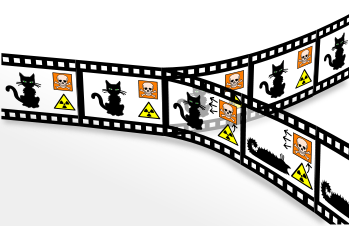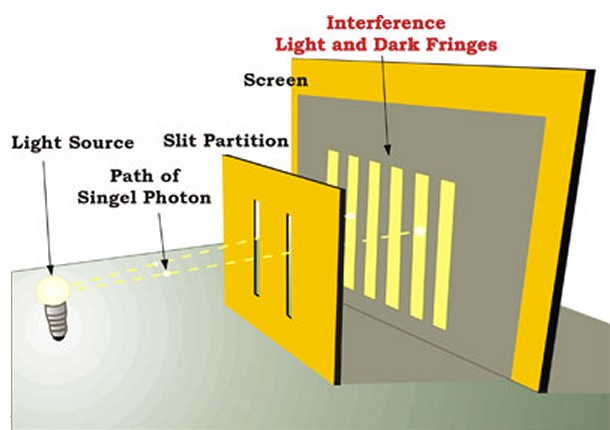# Entropy There are many, many, many worlds branching out at each moment you become aware of your environment and then make a choice ― Kevin Michel, Moving Through Parallel Worlds To Achieve Your Dreams

08 April 2015

Like a novel by Borges.

The main theme of the book Programming the Universe is that every physical system can record and process information. Understanding how the computing Universe works, is the way to understand why the universe is complex.

In the previous post, I made a short mention about Thermodynamics and the physical concept of entropy:

• Thermodynamics: the study of heat and work

• Entropy: a measure of disorder present in a physical system. A measure of the information recorded by each atom that constitutes the matter

• First Law of Thermodynamics: it’s about energy. The law states that energy is preserved when the mechanical energy is converted into heat

• Second Law of Thermodynamics: it is about information and how it is processed at a microscopic scale. The law states that the entropy (which is a measure of information) never decreases

From a computational point of view, we can say that every physical system is composed of bits which belong to two different categories:

1. invisible information (entropy), to which we have no access

2. visible information (free energy), to which we have access

The number of bits never decreases.

Entropy The word entropy (from the ancient greek ἐν en, in, and τροπή Trope, transformation) is a quantity which is interpreted as a measure of disorder. The entropy of a system is the amount of heat that can not be converted into mechanical energy. This definition was given by Rudolf Clausius in 1865. In the late nineteenth century, the founders of statistical mechanics Maxwell, Boltzmann and Gibbs realized that the entropy can be seen as a form of energy. Entropy is a measure of the number of bits of information not available recorded from atoms and molecules that make up the world. This tells us that the laws of nature preserve the information, since the bits are not destroyed. Atoms, molecules, and any other physical system in finite volume and finite energy have a finite amount of information, and then a finite number of bits.

###What information has to do with atoms?

To understand this, consider the atomic hypothesis. The ancient Greeks postulated that all matter was composed of atoms (atom means indivisible). The atomic hypothesis was based on a fundamental assumption: the refusal of infinity.

It is interesting to provide a definition of the temperature in terms of energy and entropy. The oscillation of the atoms is associated to entropy; faster oscillation require higher entropy. The temperature is a trade off between information and energy; atoms at high temperatures require more energy to record a bit. The temperature is therefore energy per bit.

###Information is preserved The laws of physics preserve information. This is called the principle of Landauer. The number of bits recorded by a system never decrease. In absence of interaction with another physical system, the amount of energy remains always the same. But how can the entropy (a form of energy) increase, without increasing the amount of information present in a system? Entropy can be intended as a quantity that measures how much energy is useful. The energy, in particular, degrades to a useful form to a form useless. There is a phenomenon in nature that can be called “the spread of ignorance.” The unknown bits infect those known. The entropy is nothing more than an information at a macroscopic level of the degree of oscillation of the particles.

Suppose that an unknown bit of information interacts with a bit of information known. After the interaction, the first bit is still unknown, but now also the second is. The unknown bit has infected the known bit, spreading the amount of ignorance and increasing the entropy of the system.

Consider an atom in a gas: its position and velocity record information. Atoms register bits. When two atoms collide, the information registered is processed. Collisions are equivalent to logical operations: AND, OR, NOT and COPY.

###Many-worlds interpretation In the classical interpretation of quantum mechanics, the so called Copenhagen interpretation, if you measure a particle to check if it is spinning is clockwise or counterclockwise, the particle will assume a direction or the other one with equal probability, and then it will evolve into one of the two possible choices.

In a Many-worlds interpretation, things are different: every time that a measurement reveals information about the state of the world in one way or another, the world splits into two and takes both paths.######Many-worlds interpretation (Credit: http://en.wikipedia.org)

Quantum mechanics is like a novel by Borges. But its quirks, as they are often defined, accurately reflect the fundamental structures of the Universe. Niels Bohr used quantum mechanics to explain successfully the structure of hydrogen atom, but as his contemporaries (Einstein among them), he was sceptical about this so counterintuitive theory.

The starting point for understanding the quantum mechanics in an intuitive level (or perhaps I should say at counterintuitive level), is to understand the particle-wave duality. The wave-particle duality reflects the fact that things we normally think as waves, like light or sound, are actually made up of particles: photons and phonons.

From Newton to Maxwell, several experiments confirmed the wave nature of light. In the late nineteenth century, Max Planck analyzed the light emitted from a stove that was heated until it becomes red. This kind of light is called black body radiation. Black objects absorb and emit light at any frequency. Planck emphasized that if light is made of waves, then the amount of energy and entropy of the radiation emitted from such kind of hot body should be infinite: a serious problem for both the first and the second Law of Thermodynamics. He was able to solve the problem by assuming that light was made of particles whose energy was proportional to the frequency of the waves. He called these particles photons, and each photon carries a finite amount of energy E.

The things that we think are waves behave like particles, and particles behave like waves. In fact, each particle has a wave associated with it, as was demonstrated by De Broglie in his doctoral thesis. Is more likely to find the particle where the wave is bigger. The distance between the wave peaks is related to the velocity of the particle. The shorter the distance the higher the speed.

The double-slit experiment proves the wave nature of particles. If a wall with two slits is illuminated with a beam of light, on the other side of the wall appears a series of black and white stripes. This is called an interference pattern. The same experiment can be repeated with particles (e.g. firing electrons and placing on the wall’s surfave a photographic plate for detection). On the slab will appear interference pattern, as if electrons were waves. The interference is a wave phenomenon. The experiments reveal that an electron behaves like a wave. You can be in two different places at the same time. Because of this wave nature a particle can be ‘here’ and ‘the’ simultaneously.######Double-slit experiment (Credit: http://www.physicsoftheuniverse.com)

And it is precisely this ability of the objects to be in many places at the same time, is responsible for the power of quantum computing. But if the particles can be in two places at the same time, and we are made of particles, then why don’t we see objects and people in different places?

The answer is that big objects tend to behave more classically and less quantum. The reason is not so much the size of the object itself, as its visibility. The larger the object, the more will be interactions that it tend to have with its environment, so it will be easier to detect. To switch between both slits simultaneously, a particle must be undetectable.

The localization process of a wave is called collapse of the wave function. When a detector is observing a specific slit, the particle must necessarily go from one side or the other, cannot pass through the both splits. And since the wave associated with the particle is no longer along the two slots, it can no longer interfere with itself and produce a pattern of light and dark. The observation (or measurement as it is often called) destroys the interference.

###Further Information

Programming the Universe, by Seth Lloyd

Ultimate physical limits to computation, by Seth Lloyd

The Universe as Quantum Computer, by Seth Lloyd

Quantum Computing, Wikipedia.org

The Road to Reality: A Complete Guide to the Laws of the Universe, by Roger Penrose

Dance of the Photons: From Einstein to Quantum Teleportation

Laws of thermodynamics, Wikipedia.org